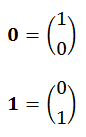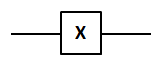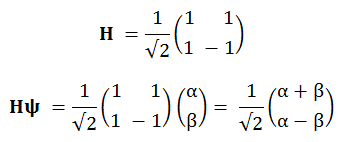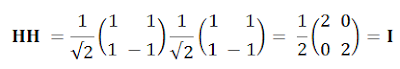## Sunday, 16 October 2011

### Quantum Computing #1: Single Qubit Gates

Previously:
Introduction
Mathematics? What Mathematics?

Recent mention of Mike Nielsen's Quantum Computation videos sparked a flurry of interest among friends and colleagues, mostly tempered by wariness about the mathematics involved. That's a pity, because there's actually very little mathematics needed to make a start on this stuff. Complex numbers, for example, are not strictly essential for an introduction to the subject. And even when they become so, well after all, they are just pairs of normal numbers, with a special rule for multiplication. Similarly, matrices are nothing more than a shorthand way of multiplying ordinary numbers pairwise, two by two, and then adding up the results.

Still it can be a sub-optimal experience to jump into Wikipedia's Quantum gate page, finding yourself immediately surrounded by not only these, but also the curious ket notation. Well, let's at least see if we can get started without that!

This is the first in a series of articles on quantum computation, to be published over the next several Sundays, loosely following the content of Prof. Nielsen's videos. It describes, in a thoroughly simplified style, a couple of single-qubit gates. Future articles will apply the same stripped-down approach to multiple qubit gates, and then measurement, and in surprisingly short order, superdense coding and quantum teleportation.

Another unusual aspect of these articles, also owed to Prof. Nielsen, is the complete lack of any reference to physical implementations of quantum gates and circuits. This is just how classical Boolean logic (or the propositional calculus) developed historically, many years before physical AND gates were realised in switches, valves, water sluices, dominoes or marbles. We simply describe a two-value quantum computational algebra, taking it on trust that there's no shortage of potentially available physical realisations.

The Computational Basis

Like a classical, logical bit, the quantum bit or qubit has two computational basis states; 0 and 1. Some writers use instead and to represent the basis states. Personally I'd have preferred to use something like T and F, but hey. Whatever symbols are used, they're usually embedded in the forementioned ket marks, like these:

but for purely typographical reasons I'll be omitting those, at least to start with, and using instead just the bold 0 and 1.

For the purposes of the matrix transformations which follow, the basis states can conveniently be represented by any two orthogonal unit column vectors. Note that the unfortunately named 0 is not the zero vector; 0 is just a label for the ordinary, basis state vector we've chosen to represent "logical zero". For example, the labels 0 and 1 might represent unit steps in some arbitrary x- and y-directions, respectively:The qubit's actual state ψ can also be any linear combination (or superposition) of these basis states,where |α|² + |β|² = 1.

Quantum Gates

Quantum computing has its own "gates", by analogy with the classical AND, OR, NOT etc. These can be represented by square matrices. For example, a single-qubit gate might be represented by a 2x2 unitary matrix M.

Wait, what? Unitary? - Well, a gate matrix multiplying any given quantum state column vector yields a new state, and this new state must also satisfy the above normalization condition, |α|² + |β|² = 1. Unitary matrices are just those which preserve this criterion. In other words, they preserve the unit length of the state vector ψ.

The simplest quantum gate is the single-qubit "wire", which - pay careful attention now - looks like this:and just transmits the state vector unaltered. The matrix representation of this is the 2x2 Identity matrix, I.The NOT Gate

For historical reasons, the quantum NOT gate is also known as the Pauli-X gate, and appears in both circuit diagrams and matrix representations as the letter X. Here's its symbol for use in quantum circuits:This gate "inverts" its input, in the sense that given an input of 0, its output will be 1; and conversely, given an input of 1, its output will be 0. As for those peculiar superposition states, it seems reasonable that an arbitrary input state ψ containing a certain quantity α of the 0 basis vector, together with a corresponding quantity β of the 1 basis vector, should upon passing through a NOT gate, have those quantities reversed. And that's just what this X does:Like the classical logical inverter, the quantum X gate is its own inverse. In terms of linear algebra it's trivial to show, by explicit matrix multiplication, that XX = I:The quantum circuit diagram below is therefore equivalent to a single wire.Our first truly quantum gate, without a classical analogue, is the Hadamard gate H. Given an input of 0, its output is the sum of the 0 and 1 basis states (normalized, of course). Similarly, given an input of 1, its output is the normalized difference between the two basis states. It's like this:The √2 is the normalization adjustment needed to give the output vector unit length. "Noise" like this is often omitted from practical quantum computations, it being universally acknowledged that the state vector always requires such normalization; but I'll retain it, to avoid confusion.

The Hadamard gate is also its own inverse. In terms of linear algebra it's trivial to show, by explicit matrix multiplication, that HH = I:and hence that the series circuit of two Hadamard gates, just like the case of two inverters, is also equivalent to a single quantum wire.

Next time: Multiple Qubit Gates.